# HSSlive: Plus One & Plus Two Notes & Solutions for Kerala State Board

## AP Board Class 7 Maths Chapter 2 Fractions Decimals and Rational Numbers Ex 3 Textbook Solutions PDF: Download Andhra Pradesh Board STD 7th Maths Chapter 2 Fractions Decimals and Rational Numbers Ex 3 Book AnswersAP Board Class 7 Maths Chapter 2 Fractions Decimals and Rational Numbers Ex 3 Textbook Solutions PDF: Download Andhra Pradesh Board STD 7th Maths Chapter 2 Fractions Decimals and Rational Numbers Ex 3 Book Answers

## Andhra Pradesh State Board Class 7th Maths Chapter 2 Fractions Decimals and Rational Numbers Ex 3 Books Solutions

 Board AP Board Materials Textbook Solutions/Guide Format DOC/PDF Class 7th Subject Maths Chapters Maths Chapter 2 Fractions Decimals and Rational Numbers Ex 3 Provider Hsslive

## How to download Andhra Pradesh Board Class 7th Maths Chapter 2 Fractions Decimals and Rational Numbers Ex 3 Textbook Solutions Answers PDF Online?

2. Click on the Andhra Pradesh Board Class 7th Maths Chapter 2 Fractions Decimals and Rational Numbers Ex 3 Answers.
3. Look for your Andhra Pradesh Board STD 7th Maths Chapter 2 Fractions Decimals and Rational Numbers Ex 3 Textbooks PDF.
4. Now download or read the Andhra Pradesh Board Class 7th Maths Chapter 2 Fractions Decimals and Rational Numbers Ex 3 Textbook Solutions for PDF Free.

## AP Board Class 7th Maths Chapter 2 Fractions Decimals and Rational Numbers Ex 3 Textbooks Solutions with Answer PDF Download

Find below the list of all AP Board Class 7th Maths Chapter 2 Fractions Decimals and Rational Numbers Ex 3 Textbook Solutions for PDF’s for you to download and prepare for the upcoming exams:

Question 1.
Find each of the following products.
(i) 56×711
(ii) 6×15
(iii) 213×315
Solution: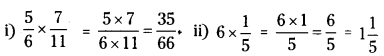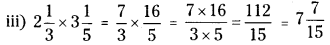Question 2.
Multiply and reduce to lowest form.
(i) 23×515
(ii) 27×13
(iii) 93×55
Solution: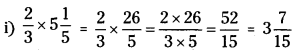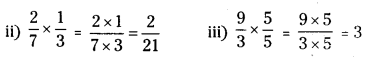Question 3.
Which one is greater’?
(i) 25 of 47 or 34 of 12
(ii) 12 of 47 or 23 of 37
Solution:
(i) 25 of 47 or 34 of 12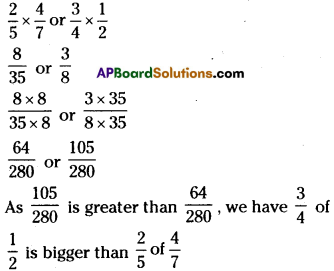(ii) 12 of 47 or 23 of 37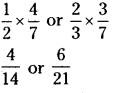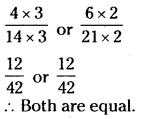Question 4.
Rehana works 212 hours each day on her embroidery. She completes the work in 7 days.
How many hours did she take to complete her work?
Solution:
Number of hours Rehana worked each day = 212 hrs
Number of days Rehana worked on embroidery = 7 days
Total time worked = 2½ x 7 = 52 x 7 = 5×72=352 = = 1712 hrs.

Question 5.
A truck runs 8 km using 1 litre of petrol. How much distance will it cover using 1023 litres of petrol?
Solution:
bistance covered wIth 1 litre of petrol 8 km
∴ Distance covered for 1023 litres petrol = 1023 x 8
= 323×8=32×83=2563=8513km.

Question 6.
Raja walks 112 meters in 1 second. How much distance will he walk in 15 minutes?
Solution:
Distance covered by Raja in 1 second = 112 m
Distance covered by Raja in 15 minutes 15 x 60 x 112
= 900 x 32 = 900×321 =1350 m

Question 7.
Provide the number in the box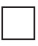to make the statement true.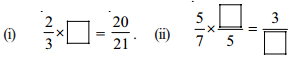## Andhra Pradesh Board Class 7th Maths Chapter 2 Fractions Decimals and Rational Numbers Ex 3 Textbooks for Exam Preparations

Andhra Pradesh Board Class 7th Maths Chapter 2 Fractions Decimals and Rational Numbers Ex 3 Textbook Solutions can be of great help in your Andhra Pradesh Board Class 7th Maths Chapter 2 Fractions Decimals and Rational Numbers Ex 3 exam preparation. The AP Board STD 7th Maths Chapter 2 Fractions Decimals and Rational Numbers Ex 3 Textbooks study material, used with the English medium textbooks, can help you complete the entire Class 7th Maths Chapter 2 Fractions Decimals and Rational Numbers Ex 3 Books State Board syllabus with maximum efficiency.

## FAQs Regarding Andhra Pradesh Board Class 7th Maths Chapter 2 Fractions Decimals and Rational Numbers Ex 3 Textbook Solutions

#### Can we get a Andhra Pradesh State Board Book PDF for all Classes?

Yes you can get Andhra Pradesh Board Text Book PDF for all classes using the links provided in the above article.

## Important Terms

Andhra Pradesh Board Class 7th Maths Chapter 2 Fractions Decimals and Rational Numbers Ex 3, AP Board Class 7th Maths Chapter 2 Fractions Decimals and Rational Numbers Ex 3 Textbooks, Andhra Pradesh State Board Class 7th Maths Chapter 2 Fractions Decimals and Rational Numbers Ex 3, Andhra Pradesh State Board Class 7th Maths Chapter 2 Fractions Decimals and Rational Numbers Ex 3 Textbook solutions, AP Board Class 7th Maths Chapter 2 Fractions Decimals and Rational Numbers Ex 3 Textbooks Solutions, Andhra Pradesh Board STD 7th Maths Chapter 2 Fractions Decimals and Rational Numbers Ex 3, AP Board STD 7th Maths Chapter 2 Fractions Decimals and Rational Numbers Ex 3 Textbooks, Andhra Pradesh State Board STD 7th Maths Chapter 2 Fractions Decimals and Rational Numbers Ex 3, Andhra Pradesh State Board STD 7th Maths Chapter 2 Fractions Decimals and Rational Numbers Ex 3 Textbook solutions, AP Board STD 7th Maths Chapter 2 Fractions Decimals and Rational Numbers Ex 3 Textbooks Solutions,
Share: